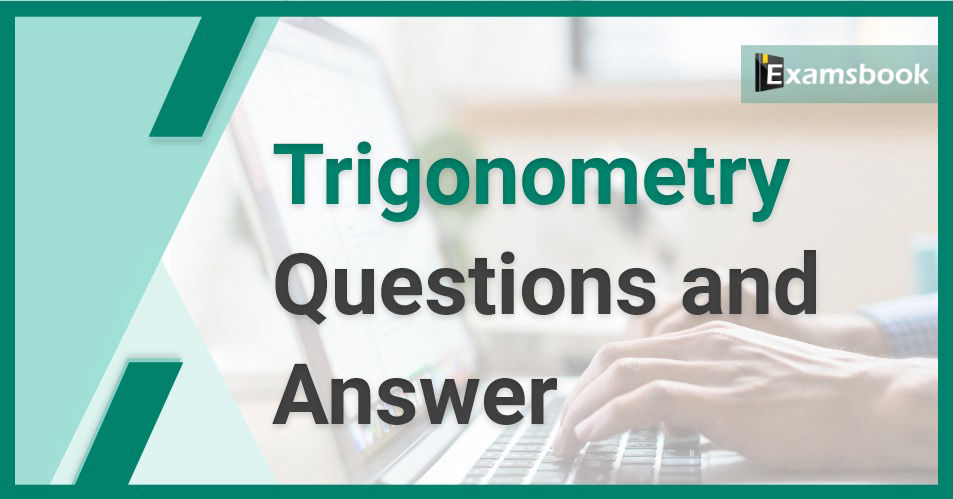• Save

# Trigonometry Questions and Answers for Competitive Exams

Vikram Singh9 months ago 3.0K ViewsTrigonometry is the oldest topic in the history of mathematics. This topic is mainly related to the sides of triangles and the angles of different figures. Unknown sides and angles are studied using trigonometry formulas or trigonometric identities. In trigonometry, angles are measured in degrees or radians.

The important angles are 0°, 30°, 45°, 60°, and 90°. Don't be the fear of this topic remains in the mind of the students, we have sharing practice questions here related to Trigonometry Questions and Answers for the competitive exams. Start your practice below with some important questions for you.

## Trigonometry Maths Questions

Q :

(Cos 0°+Sin 45° +Sin 30°)(Sin 90°-Cos 45°+ Cos 60°) is equal to:

(A) $${7\over 4}$$

(B) $${5\over 8}$$

(C) $${5\over 6}$$

(D) $${3\over 5}$$

Q :

ΔPQR is right angled at Q. If cos P = 3/5, then what is the value of cos R?

(A) $${3\over4}$$

(B) $${5\over3}$$

(C) $${4\over5}$$

(D) $${4\over3}$$

Q :

If sec x + cos x = 2, then the value of sec16 x + cos16 x will be-

(A) 2

(B) 0

(C) 1

(D) √3

Q :

Simplify $$({Sinθ\over{1+cosθ}}+{1+cosθ\over sinθ})({1\over (tanθ+cotθ)})$$

(A) $${cos{\ θ}}$$

(B) $${2sin{\ θ}}$$

(C) $${sin{\ θ}}$$

(D) $${2cos{\ θ}}$$

Q :

If Cos A + Cos2 A = 1, then Sin2 A + Sin4 A=?

(A) 0

(B) 1

(C) $${1\over 2}$$

(D) -1

Q :

If cosec θ=1.25 then $${4 \ tanθ-5 \ cosθ + 1\over secθ+ 4cotθ-1}=?$$

(A) $${9\over 10}$$

(B) 2

(C) $${10\over 11}$$

(D) $${1\over 2}$$

Q :

One angle of a triangle is 55°. If the other two angles are in the ratio 9:16, find the angles?

(A) 65° और 115°

(B) 90° और 160°

(C) 55°और 165°

(D) 45° और 80°

Q :

If a=tanθ + 1 and b=sinθ+cosθ then find (b2-1)a=?

(A) ab

(B) 2bsecθ

(C) 2b

(D) 2bsin θ

Q :

If $${sin\ {θ}}={1\over {\sqrt{2}}}$$ then (tanθ+cosθ)=?

(A) $${1\over{\sqrt{2}}}$$

(B) $${2\over{\sqrt{2}}}$$

(C) $${3\over{\sqrt{2}}}$$

(D) $${(1+\sqrt{2})\over{\sqrt{2}}}$$

Q :

If 1 + sin2 A = 3 sin A cos A, then what are the possible values of tan A?

(A) 1, 0

(B) 1, 1/2

(C) 1 , 1/3

(D) 1, 3

### Vikram Singh

Providing knowledgable questions of Reasoning and Aptitude for the competitive exams.